### 13.1.3.4 Trapped on a surface

It is possible that the constraints cause the configuration to be trapped on a lower dimensional surface. Let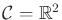, and consider the system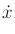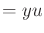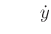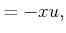(13.22)

for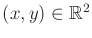and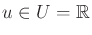. What are the integral curves for a constant action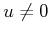? From any point, the trajectory follows a circle of radius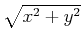centered at the origin. The speed along the circle is determined by, and the direction is determined by the sign of. Therefore, (13.22) indicates that the configuration is confined to a circle. Other than that, there are no further constraints.

Suppose that the initial configuration is given as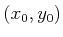. Since the configuration is confined to a circle, the C-space could alternatively be defined as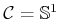. Each point on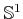can be mapped to the circle that has radius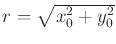and center at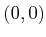. In this case, there are no differential constraints on the velocities, provided that motions are trapped on the circle. Any velocity in the one-dimensional tangent space at points on the circle is allowed. This model is equivalent to (13.22).

Now consider the possible trajectories that are constrained to traverse a circle,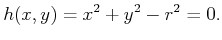(13.23)

This means that for all time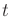,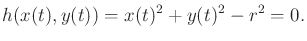(13.24)

To derive a constraint on velocities, take the derivative with respect to time, which yields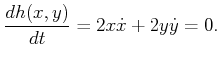(13.25)

This is an example of a Pfaffian constraint, as given in (13.5). The parametric form of this differential constraint happens to be (13.22). Any velocity vector that is a multiple of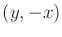satisfies (13.25). When expressed as a differential constraint, the radius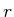does not matter. This is because it is determined from the initial configuration.

What just occurred here is a special case of a completely integrable differential model. In general, if the model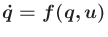can be expressed as the time derivative of constraints of the form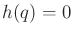, then the configuration transition equation is said to be completely integrable. Obtaining an implicit differential model from constraints of the form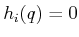is not difficult. Each constraint is differentiated to obtain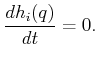(13.26)

For example, such constraints arise from closed kinematic chains, as in Section 4.4, and the implicit differential model just expresses the condition that velocities must lie in the tangent space to the constraints. It may be difficult, however, to obtain a parametric form of the differential model. Possible velocity vectors can be computed at any particular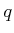, however, by using the linear algebra techniques described in Section 7.4.1.

It is even quite difficult to determine whether a differential model is completely integrable, which means that the configurations are trapped on a lower dimensional surface. For some systems, to be described by (13.41), this will be solved by the Frobenius Theorem in 15.4.2. If such systems are not completely integrable, they are called nonholonomic; otherwise, they are called holonomic. In general, even if a model is theoretically integrable, actually performing the integration is another issue. In most cases, it is difficult or impossible to integrate the model.

Therefore, it is sometimes important to work directly with constraints in differential form, even if they are integrable. Furthermore, methods for planning under differential constraints can be applied to problems that have constraints of the form. This, for example, implies that motion planning for closed kinematic chains can be performed by planning algorithms designed to handle differential constraints.

Steven M LaValle 2020-08-14